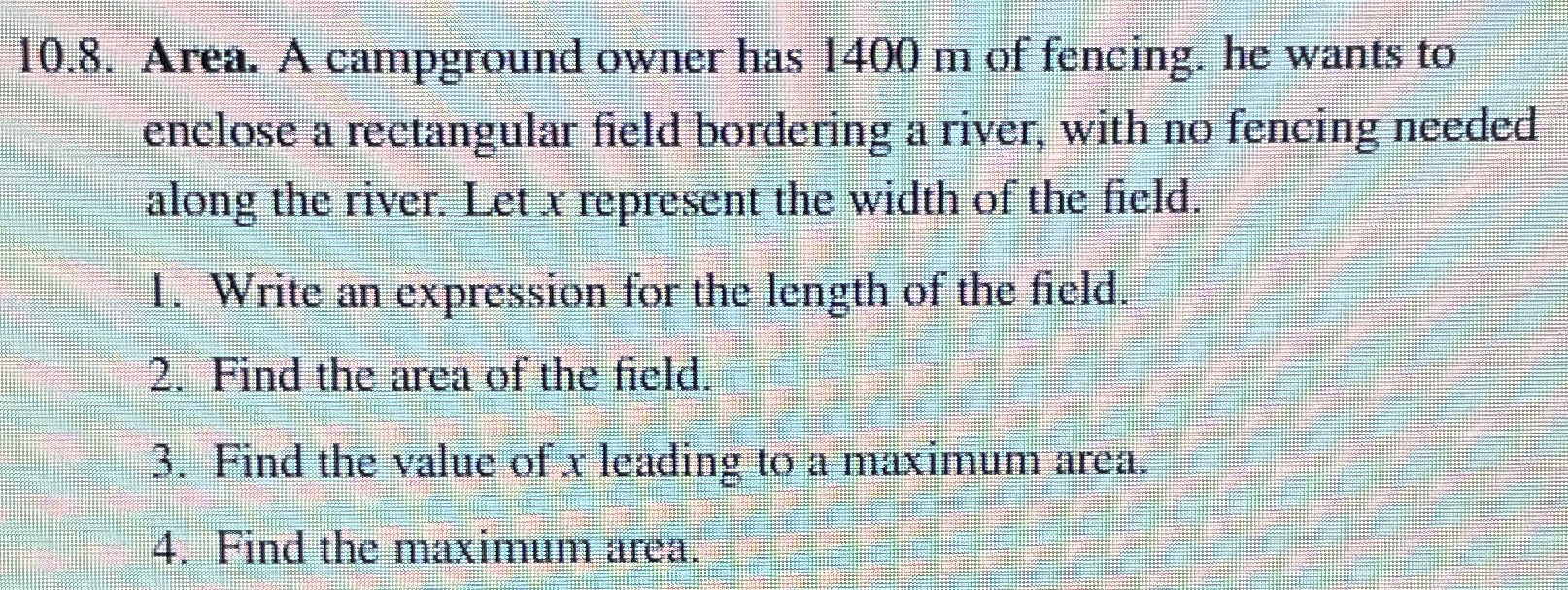### ¿Todavía tienes preguntas de matemáticas?

Pregunte a nuestros tutores expertos
Algebra
Pregunta

A campground owner has $$1400 m$$ of fencing. he wants to enclose a rectangular field bordering a river, with no fencing needed along the river. Let $$x$$ represent the width of the field.

1. Write an expression for the length of the field.

2. Find the area of the field.

3. Find the value of $$x$$ leading to a maximum area.

4. Find the maximum area.2.1400x-2x$$^{2}$$